## Estimating Transfer Function Models for a Heat Exchanger

This example shows how to estimate a transfer function from measured signal data.

### Heat Exchanger

In this example we estimate the transfer function for a heat exchanger. The heat exchanger consists of a coolant temperature, product temperature, and disturbance ambient temperature. We will estimate the coolant to product temperature transfer function.

### Configuring the Measured Data

The measured data is stored in a MATLAB file and includes measurements of changes in coolant temperature around a nominal and changes in product temperature around a nominal.

```load iddemo_heatexchanger_data ```

Collect the measured data using the `iddata` command and plot it.

```data = iddata(pt,ct,Ts); data.InputName = '\Delta CTemp'; data.InputUnit = 'C'; data.OutputName = '\Delta PTemp'; data.OutputUnit = 'C'; data.TimeUnit = 'minutes'; plot(data) ```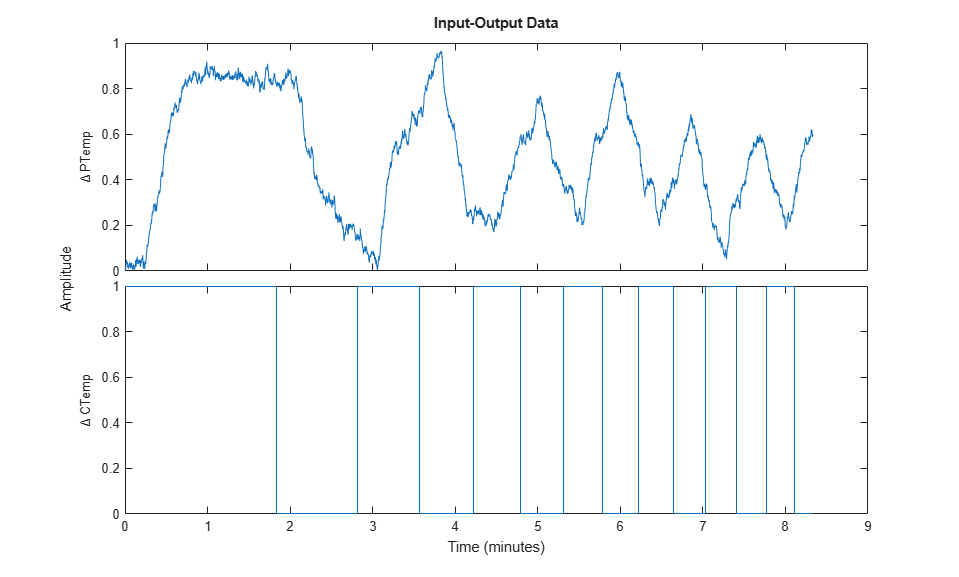### Transfer Function Estimation

From the physics of the problem we know that the heat exchanger can be described by a first order system with delay. Use the `tfest` command specifying one pole, no zeroes, and an unknown input/output delay to estimate a transfer function.

```sysTF = tfest(data,1,0,nan) ```
```sysTF = From input "\Delta CTemp" to output "\Delta PTemp": 1.467 exp(-0.0483*s) * -------- s + 1.56 Continuous-time identified transfer function. Parameterization: Number of poles: 1 Number of zeros: 0 Number of free coefficients: 2 Use "tfdata", "getpvec", "getcov" for parameters and their uncertainties. Status: Estimated using TFEST on time domain data "data". Fit to estimation data: 36% FPE: 0.02625, MSE: 0.02622 ```

The `compare` and `resid` commands allow us to investigate how well the estimated model matches the measured data.

```set(gcf,'DefaultAxesTitleFontSizeMultiplier',1,... 'DefaultAxesTitleFontWeight','normal',... 'Position',[100 100 780 520]); resid(sysTF,data); ``````clf compare(data,sysTF) ```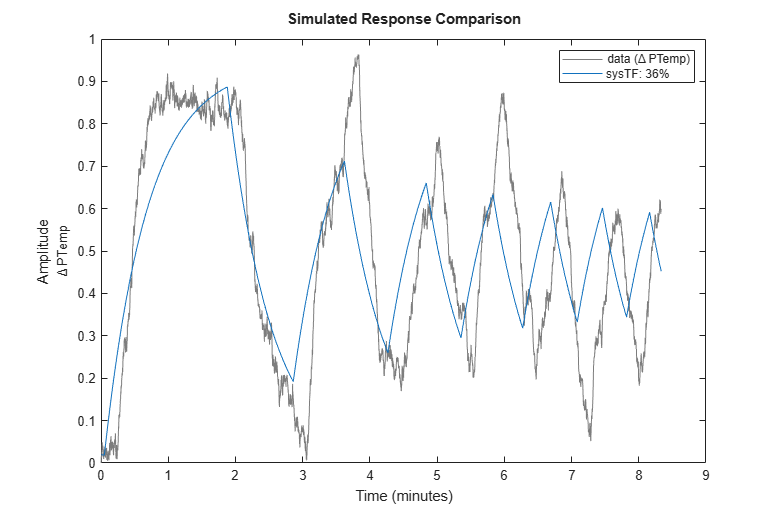The figure shows that residuals are strongly correlated implying there is information in the measured data that has not been adequately captured by the estimated model.

### Transfer Function Estimation from an Initial System

Previously we estimated a transfer function from data but apart for the system order did not include much apriori knowledge. From the physics of the problem we know that the system is stable and has positive gain. Inspecting the measured data we also know that the input/output delay is around 1/5 of a minute. We use this information to create an initial system and estimate a transfer function using this system as the initial guess.

```sysInit = idtf(NaN,[1 NaN],'ioDelay',NaN); sysInit.TimeUnit = 'minutes'; ```

Restrict the transfer function numerator and denominator terms so that the system is stable with positive gain.

```sysInit.Structure.num.Value = 1; sysInit.Structure.num.Minimum = 0; sysInit.Structure.den.Value = [1 1]; sysInit.Structure.den.Minimum = [0 0]; ```

Restrict the input/output delay to the range [0 1] minute and use 1/5 minute as an initial value.

```sysInit.Structure.ioDelay.Value = 0.2; sysInit.Structure.ioDelay.Minimum = 0; sysInit.Structure.ioDelay.Maximum = 1; ```

Use the system as an initial guess for the estimation problem

```sysTF_initialized = tfest(data,sysInit) ```
```sysTF_initialized = From input "\Delta CTemp" to output "\Delta PTemp": 1.964 exp(-0.147*s) * --------- s + 2.115 Continuous-time identified transfer function. Parameterization: Number of poles: 1 Number of zeros: 0 Number of free coefficients: 2 Use "tfdata", "getpvec", "getcov" for parameters and their uncertainties. Status: Estimated using TFEST on time domain data "data". Fit to estimation data: 54.09% FPE: 0.01351, MSE: 0.01349 ```
```resid(sysTF_initialized,data); ```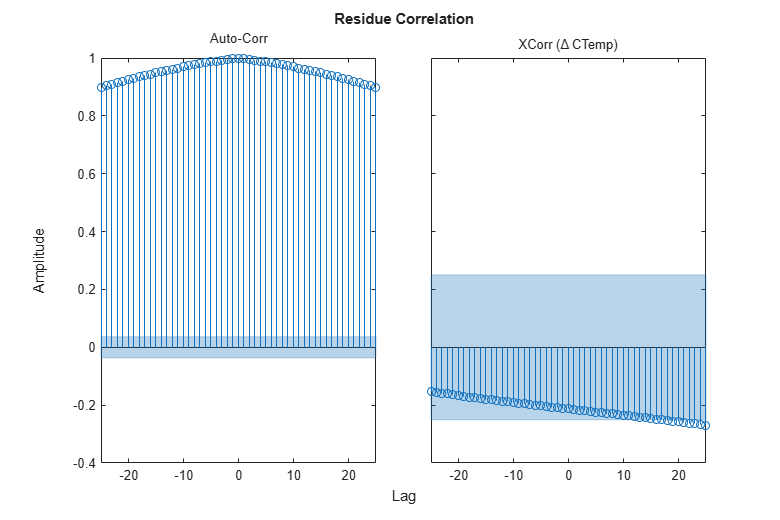```clf compare(data,sysTF,sysTF_initialized) ```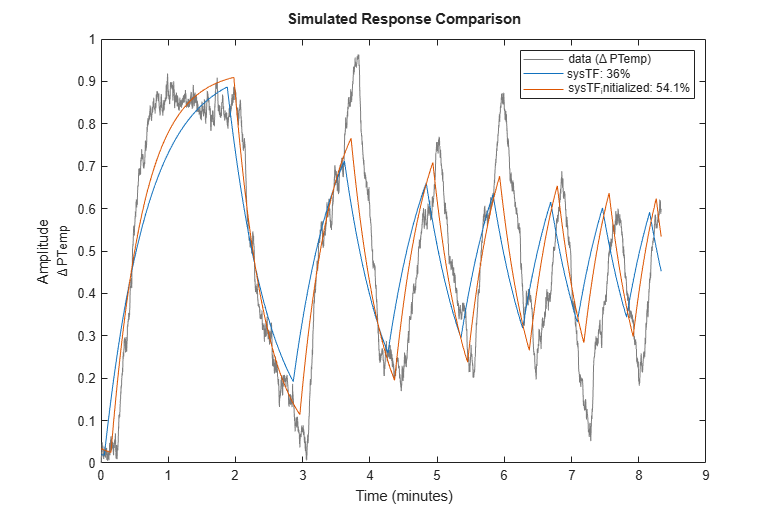### Process Model Estimation

In the above we treated the estimation problem as a transfer function estimation problem, but we know that there is some additional structure we can impose. Specifically the heat exchanger system is known to be a 1st order process with delay, or 'P1D' model of the form:

``

Use the `procest` command to further impose this structure on the problem.

```sysP1D = procest(data,'P1D') ```
```sysP1D = Process model with transfer function: Kp G(s) = ---------- * exp(-Td*s) 1+Tp1*s Kp = 0.90548 Tp1 = 0.32153 Td = 0.25435 Parameterization: 'P1D' Number of free coefficients: 3 Use "getpvec", "getcov" for parameters and their uncertainties. Status: Estimated using PROCEST on time domain data "data". Fit to estimation data: 70.4% FPE: 0.005614, MSE: 0.005607 ```
```resid(sysP1D,data); ```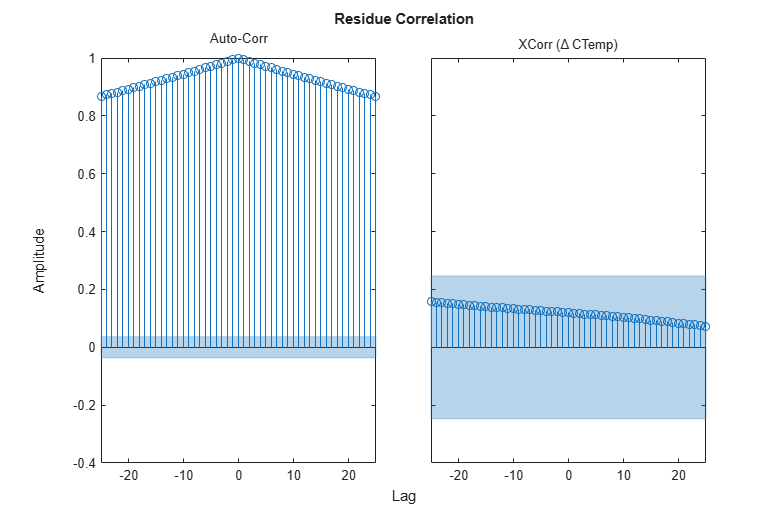```clf compare(data,sysTF,sysTF_initialized,sysP1D) ```### Process Model Estimation with Disturbance Model

The residual plots of all the estimations performed so far show that the residual correlation is still high, implying that the model is not rich enough to explain all the information in the measured data. The key missing piece is the disturbance ambient temperature which we have not yet included in the model.

Create a 'P1D' process model with restriction on delay and time constant values and use this as the initial guess for the estimation problem.

```sysInit = idproc('P1D','TimeUnit','minutes'); ```

Restrict the model to have positive gain, and delay in the range [0 1] minute.

```sysInit.Structure.Kp.Value = 1; sysInit.Structure.Kp.Minimum = 0; sysInit.Structure.Tp1.Value = 1; sysInit.Structure.Tp1.Maximum = 10; sysInit.Structure.Td.Value = 0.2; sysInit.Structure.Td.Minimum = 0; sysInit.Structure.Td.Maximum = 1; ```

Specify the option to use a first-order model ('ARMA1') for the disturbance component. Use the template model `sysInit` along with the option set to estimate the model.

```opt = procestOptions('DisturbanceModel','ARMA1'); sysP1D_noise = procest(data,sysInit,opt) ```
```sysP1D_noise = Process model with transfer function: Kp G(s) = ---------- * exp(-Td*s) 1+Tp1*s Kp = 0.91001 Tp1 = 0.3356 Td = 0.24833 An additive ARMA disturbance model has been estimated y = G u + (C/D)e C(s) = s + 591.6 D(s) = s + 3.217 Parameterization: 'P1D' Number of free coefficients: 5 Use "getpvec", "getcov" for parameters and their uncertainties. Status: Estimated using PROCEST on time domain data "data". Fit to estimation data: 96.86% (prediction focus) FPE: 6.307e-05, MSE: 6.294e-05 ```
```resid(sysP1D_noise,data); ```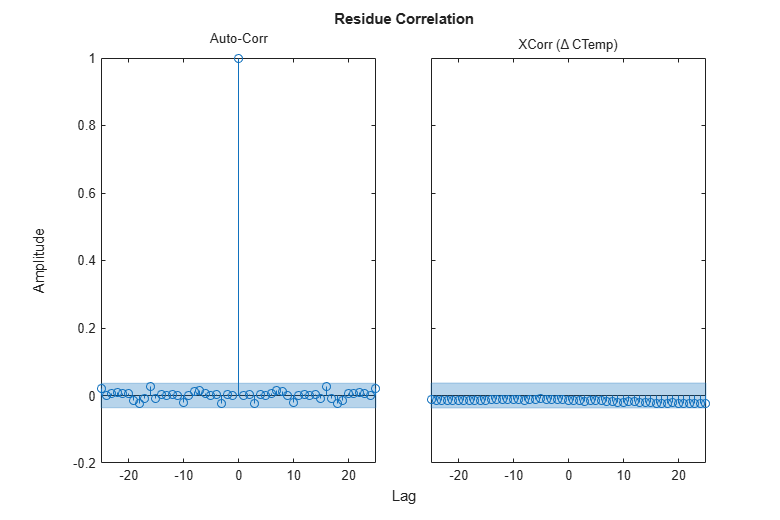```clf compare(data,sysTF,sysTF_initialized,sysP1D,sysP1D_noise) ```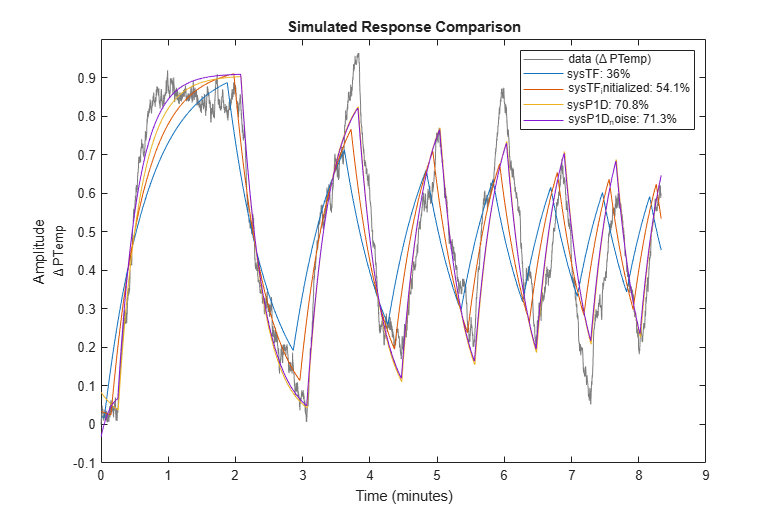The residual plot clearly shows that the residuals are uncorrelated implying that we have a model that explains the measured data. The 'ARMA1' disturbance component we estimated is stored as numerator and denominator values in the "NoiseTF" property of the model.

```sysP1D_noise.NoiseTF ```
```ans = struct with fields: num: {[1 591.6038]} den: {[1 3.2172]} ```

### Compare Different Models

Although we have identified a model that explains the measured data we note that the model fit to measured data is around 70%. The loss in fit value is a consequence of the strong effect of the ambient temperature disturbance which is illustrated as follows.

The measured data was obtained from a Simulink model with the following exact values (d is a Gaussian noise disturbance)

` y = (1-pi/100)*exp(-15s)/(21.3s+1)*u + 1/(25s+1)*d`

Create a 'P1D' model with these values and see how well that model fits the measured data.

```sysReal = idproc('P1D','TimeUnit','minutes'); sysReal.Kp = 1-pi/100; sysReal.Td = 15/60; sysReal.Tp1 = 21.3/60; sysReal.NoiseTF = struct('num',{[1 10000]},'den',{[1 0.04]}); ```
```compare(data,sysReal,sysTF,sysTF_initialized,sysP1D,sysP1D_noise); ```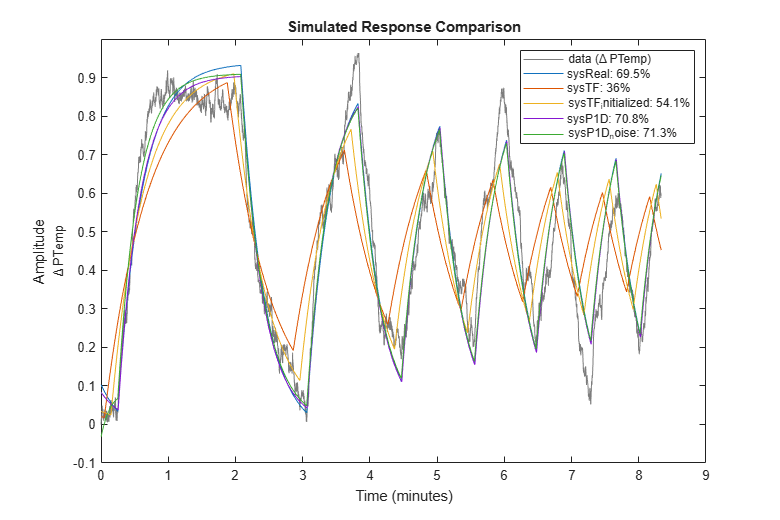The comparison plot shows that the true system fit to measured data is also around 70% confirming that our estimated models are no worse than the real model in fitting measured data - a consequence of the strong effect of the ambient temperature disturbance.